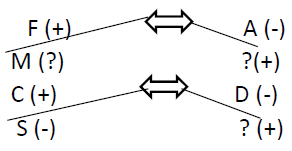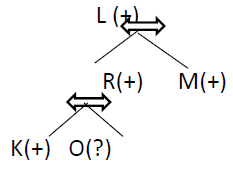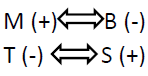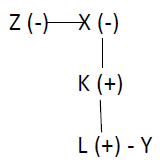# Reasoning - Coded Relationship

Coded relationship contains problems that involve interpreting a given relationship-string, which is coded in a particular fashion and then matching it with the relationship mentioned in the question.

We know that such problems involve interpreting a given relationship-string which is coded in a particular fashion. The process of decoding each and every relation and then interpreting from the given relationship-string, is the final process which is a cumbersome process and doing all of it for all the choices makes it very time taking. Hence, following are some of the shortcuts −

Step I − Eliminate all wrong choices by check sex and check generation gap method.

### Check Sex

In some questions, if you analyse symbols, you can easily find that the person you are considering is a male. For example, if ‘A + B’ means ‘A’ is the father of ‘B’, then ‘A + B × C – D’ implies that A is the father and therefore must be a male, i.e. A should be followed by ‘+’. Therefore, all the choices that mention A as sister/daughter/wife, etc. must be wrong.

### Check Generation Gap

Sometimes, it may be time taking to actually find out the relation between two persons. But a rough estimate of the generation gap can be easily found out. For this, you should assume that −

• Mother/father to son or daughter have a generation gap of +1.
• Son/daughter to mother/father has a generation gap of -1.
• Brothers/sisters and husband/wife have a generation gap of zero.

Using this we can easily find out the generation gap between the persons under consideration and eliminate the wrong choices.

Example

Suppose −

‘M Δ N’ means ‘M is mother of N’

‘M • N’ means ‘M is sister of N’

‘M ∗ N’ means ‘M is father of N’

‘M β N’ means ‘M is brother of N’

Then which of the following means ‘L is paternal grandfather of O’?

Option 1 − L ∗ R • M β K β O

Option 2 − R ∗ L Δ P β K • O

Option 3 − L ∗ M Δ R ∗ β O

Option 4 − L ∗ R β M ∗ K β O

Answer − ‘Δ’ and ‘∗’ are mother and father relations. So, each of them implies a generation gap of +1. Similarly, ‘•’ and ‘β’ imply a generation gap of zero as shown in the following chart.

 Symbol Generation Gap Δ +1 • 0 ∗ +1 β 0

So, generation gap in choice 1 is L (+1) R (0) M (0) K (0) O = +1

For choice 3, L (+1) M (+1) R (+1) K (0) O = +3

But, the generation gap between grandfather and grandchildren should be +2. Therefore, both choices are wrong. Again choice 2 is wrong because, here ‘L’ is mother of ’P’. It doesn’t obey check sex rule. Hence this leaves us with choice 4.

Step II − Draw a family-tree for remaining choices.

Please remember that using our earlier step, we only get some help in eliminating some wrong answers quickly. But we don’t arrive at the correct answer. To decide exactly the correct choice from the remaining options, you will need to draw a family tree.

ExampleThis diagram tells us that −

• F and A are a couple, F is the husband while A is the wife.

• F has a sister K.

• The couple F and A has three children: M, C, and another son, whose name is not known. C is also a son while the sex of M is not known.

• M and other unknown son are unmarried while C is married to D.

• The couple, C and D has a daughter S and a son whose name is not known.

So, from the previous example we have two options left. Let’s draw a family tree.Example

‘A + B’ means ‘A is daughter of B’; ‘A × B’ means ‘A is the son of B’ and ‘A – B’ means ‘A is the wife of B’. If ‘T – S × B – M’, which of the following is not true?

• B is mother of S
• M is husband of B
• T is wife of S
• S is daughter of B
• S is son of B

Here, ‘check sex’ and ‘generation gap’ are not needed. Only the family tree is enough to know the answers.From the above we find, B is mother of S. M is husband of B. T is wife of S. S is son of B. Hence S is the son of B statement is true.

### Quicker Method

Consider choice (4) and choice (5), S can be either a son or daughter of B. So, one of these choices must be false. But in the given statement, immediately after S we have a ‘×’ sign. Therefore, this sign must be a male. Hence choice (4) is false. Choice (5) is correct.

Basically, there are two types of coding.

• Backward Type
• Forward Type

### Backward-type and Forward-type coding

‘A + B’ means ‘A is father of B’ is a forward-type coding and ‘A × B’ means ‘B is father of A’ is a backward-type coding.

So, in forward-type coding, the first person is the given relation of the second person. But in backward-type coding, the second person is the given relation of the first person.

### Calculation of Generation Gap

Step I − Take one of the people under consideration.

Step II − Move rightwards. For each father/mother relation put a ‘+1’, for each brother/sister/husband/wife relation put a ‘0’, for each son/daughter relation put a ‘-1’.

Step III − Put the sum total.

Note − The above is applicable only for forward-type coding. For backward-type coding, the process has one minor change. Here, instead of moving from the left to the right, we start from the right side. Rest methods are the same.

Example

Directions (Q. 1-2) − Read the following information to answer the questions.

• ‘P # Q’ means ‘Q is father of P’
• ‘P ∗ Q’ means ‘Q is mother of P’
• ‘P £ Q’ means ‘Q is brother of P’
• ‘P \$ Q’ means ‘Q is sister of P’

1. Which of the following means ‘X is the grandfather of Y’?

A − Z \$ X ∗ K # L £ Y

B − Y £ L # K ∗ X \$ Z

C − Y ∗ K # L £ Y

D − can’t be determined

E − None of these

Explanation − In this case, we have backward type coding. Therefore, if X is the grandmother of Y, X must come after Y in the sequence. So, choice (1) and (3) are wrong. Now, draw the family tree for choice (2).Hence X is the grandmother of Y.

2. Which of the following means ‘A is the nephew of D’?

A − D £ C # B £ A

B − A £ B # D £ C

C − C £ D # B £ A

D − Can’t be determined

E − None of these